# MCQs on Control Systems

##### Page 12 of 23. Go to page 1 2 3 4 5 6 7 8 9 10 11 12 13 14 15 16 17 18 19 20 21 22 23
01․ Sparking between the contacts can be reduced by inserting
a capacitor in series with the contacts.
a capacitor in parallel with the contacts.
a reactor in the line.
a resistor in the line.

An arc between two electrodes can be initiated by ionization of atmospheric air. If we connect a capacitor across the contacts of the electrodes, there is a chance to make a short circuit by capacitor. Hence, there is no air gap between contacts and thereby sparking can be reduced.

02․ Error constants of a system are a measure of
transient state response.
steady state response as well as transient state response.
relative stability.

In the steady state condition, the accuracy of the time response is the indication of how well the system responds achieving the final settling stage. Ideally, the output y (t) equals to the reference signal u (t) and thereby the error e (t) is zero, because error is the difference between the reference signal and the output signal. In practice, it is not possible. But, the objective is to force the output signal to reach the reference signal. For that purpose only, error constants are introduced to achieve the steady state response. So, if we adjust the error constants, then the system's steady state response also varies.

03․ Slope of asymptote in Bode plot of 2nd order system is _____________________ per octave.
18 dB
12 dB
6 dB
3 dB

Basically, the slope of an asymptote in a Bode plot of any system are n × 20 dB per decade or n × 6 dB per octave (an octave is a change in frequency by a factor of 2). Here, n is the order of the system. Hence, for a second order system, the slope is given by (2 × 6 = 12) dB per octave.

04․ The graph of an electrical network has N nodes and B branches. The number of links, L with respect to the choice of a tree, is given by
B - N + 1.
B + N.
N - B + 1.
N - 2B - 1.

For a graph with N nodes, the number of branches in each tree T must be; T = N - 1. An important relationship for circuit analysis is; B = L + T. where B is the number of branches in the graph and L is the number of links removed to form the tree. Hence, the number of links L = B - N + 1.

05․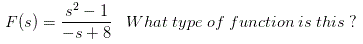It is a PR function.
It is not a PR function.
Data insufficient.
None of these.

PR function tests reveals that all the quotients of polynomial in the numerator are not +ve and also there is a zero on the r.h.s of s-plane. This clearly proves that the given function is not a PR function. No more confirmatory testing is needed.

06․ First Foster form of a LC network represents which element in the beginning?
Capacitor.
Resistor.
Both A and B.

In Foster 1 form first element is a capacitor.

07․ What is the full form of SPICE ?
Stimulated program with integrated circuit arrangement.
Simulated program with integrated circuit analysis.
Sequential program with integrated circuit analysis.
None of these.

SPICE is a software that simulates different circuits and can perform various analysis of electrical and electronics circuits.

08․ Based on relation between the arm impedances the filters can be categorized into how many types?
3.
4.
2.
Not classified at all.

2 types are - constant k type / prototype filter and m-derived filter.

09․ The transfer function of a system is given as below, if a = 0.1, then the steady state error to unity ramp input will be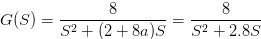0.49.
0.35.
0.25.
0.16.

Steady state error is given by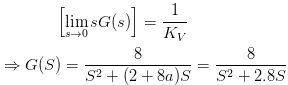∴ KV = 8/2.8; So steady state error = 1/KV = 0.35.

10․ The unit step error coefficient of a system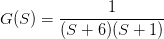with unity feedback is
0.
infinity.
1.
1/6.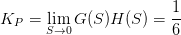<<<1011121314>>>